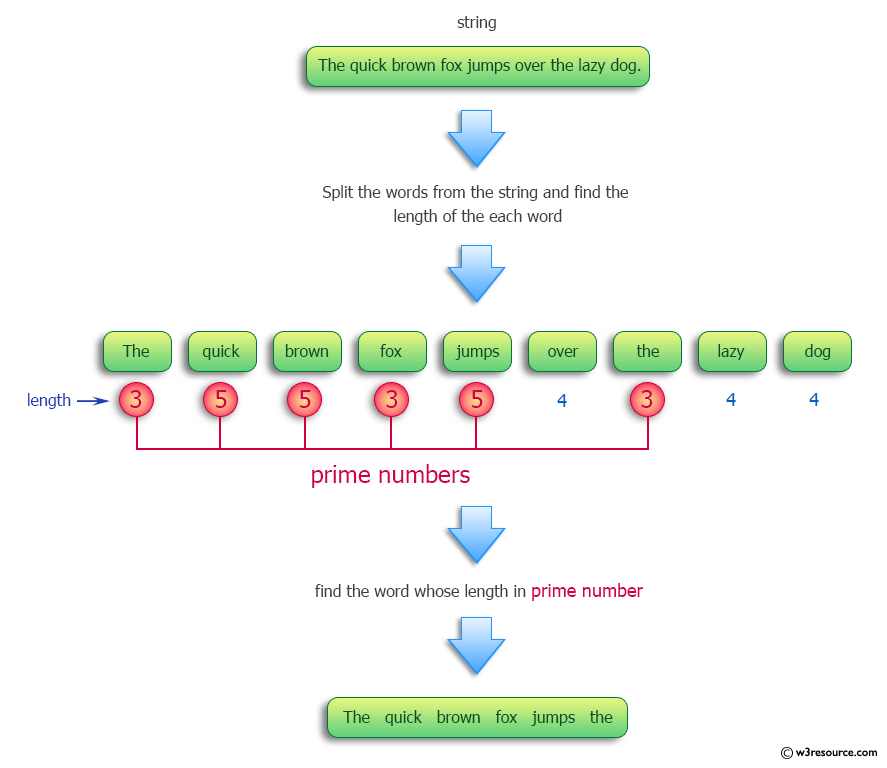﻿ Python: Find the string consisting of all the words whose lengths are prime numbers - w3resource# Python: Find the string consisting of all the words whose lengths are prime numbers

## Python Programming Puzzles: Exercise-64 with Solution

Write a Python program to find the string consisting of all the words whose lengths are prime numbers.

```Input:
The quick brown fox jumps over the lazy dog.
Output:
The quick brown fox jumps the

Input:
Omicron Effect: Foreign Flights Won't Resume On Dec 15, Decision Later.
Output:
Omicron Effect: Foreign Flights Won't On Dec 15,
```

Pictorial Presentation:Sample Solution:

Python Code:

``````#License: https://bit.ly/3oLErEI

def test(strs):
return " ".join(strs for strs in strs.split() if is_prime(len(strs)))
def is_prime(n):
return n > 1 and all(n % j for j in range(2, int(n ** 0.5) + 1))
strs = "The quick brown fox jumps over the lazy dog."
print("Original list of numbers:")
print(strs)
print("Words whose lengths are prime numbers in the said string:")
print(test(strs))
strs = "Omicron Effect: Foreign Flights Won't Resume On Dec 15, Decision Later."
print("\nOriginal list of numbers:")
print(strs)
print("Words whose lengths are prime numbers in the said string:")
print(test(strs))
``````

Sample Output:

```Original list of numbers:
The quick brown fox jumps over the lazy dog.
Words whose lengths are prime numbers in the said string:
The quick brown fox jumps the

Original list of numbers:
Omicron Effect: Foreign Flights Won't Resume On Dec 15, Decision Later.
Words whose lengths are prime numbers in the said string:
Omicron Effect: Foreign Flights Won't On Dec 15,
```

Flowchart:## Visualize Python code execution:

The following tool visualize what the computer is doing step-by-step as it executes the said program:

Python Code Editor :

Have another way to solve this solution? Contribute your code (and comments) through Disqus.

What is the difficulty level of this exercise?

Test your Programming skills with w3resource's quiz.

﻿

## Python: Tips of the Day

Clamps num within the inclusive range specified by the boundary values x and y:

Example:

```def tips_clamp_num(num,x,y):
return max(min(num, max(x, y)), min(x, y))
print(tips_clamp_num(2, 4, 6))
print(tips_clamp_num(1, -1, -6))
```

Output:

```4
-1
```